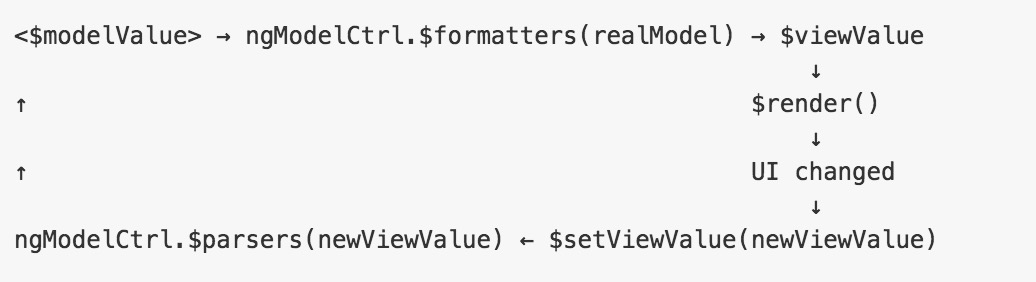# Angular Input格式化

`````` <input type="text" ng-model="demo.text | uppercase" />
````````````.directive('textTransform', [function() {

return {
require: 'ngModel',
link: function(scope, element, iAttrs, ngModelCtrl) {
ngModelCtrl.\$parsers.push(function(value) {
...
});

ngModelCtrl.\$formatters.push(function(value) {
...
});
}
};
}]);
``````

`````` .directive('textTransform', function() {
var transformConfig = {
uppercase: function(input){
return input.toUpperCase();
},
capitalize: function(input){
return input.replace(
/([a-zA-Z])([a-zA-Z]*)/gi,
function(matched, \$1, \$2){
return \$1.toUpperCase() + \$2;
});
},
lowercase: function(input){
return input.toLowerCase();
}
};
return {
require: 'ngModel',
link: function(scope, element, iAttrs, modelCtrl) {
var transform = transformConfig[iAttrs.textTransform];
if(transform){
modelCtrl.\$parsers.push(function(input) {
return transform(input || "");
});

element.css("text-transform", iAttrs.textTransform);
}
}
};
});
``````

``````<input type="text" ng-model="demo.text" text-transform="capitalize" />
<input type="text" ng-model="demo.text" text-transform="uppercase" />
<input type="text" ng-model="demo.text" text-transform="lowercase" />
``````